# 如何用霍夫变换算法实现直线检测

• 2020 年 5 月 28 日
• 本文字数：5826 字

阅读完需：约 19 分钟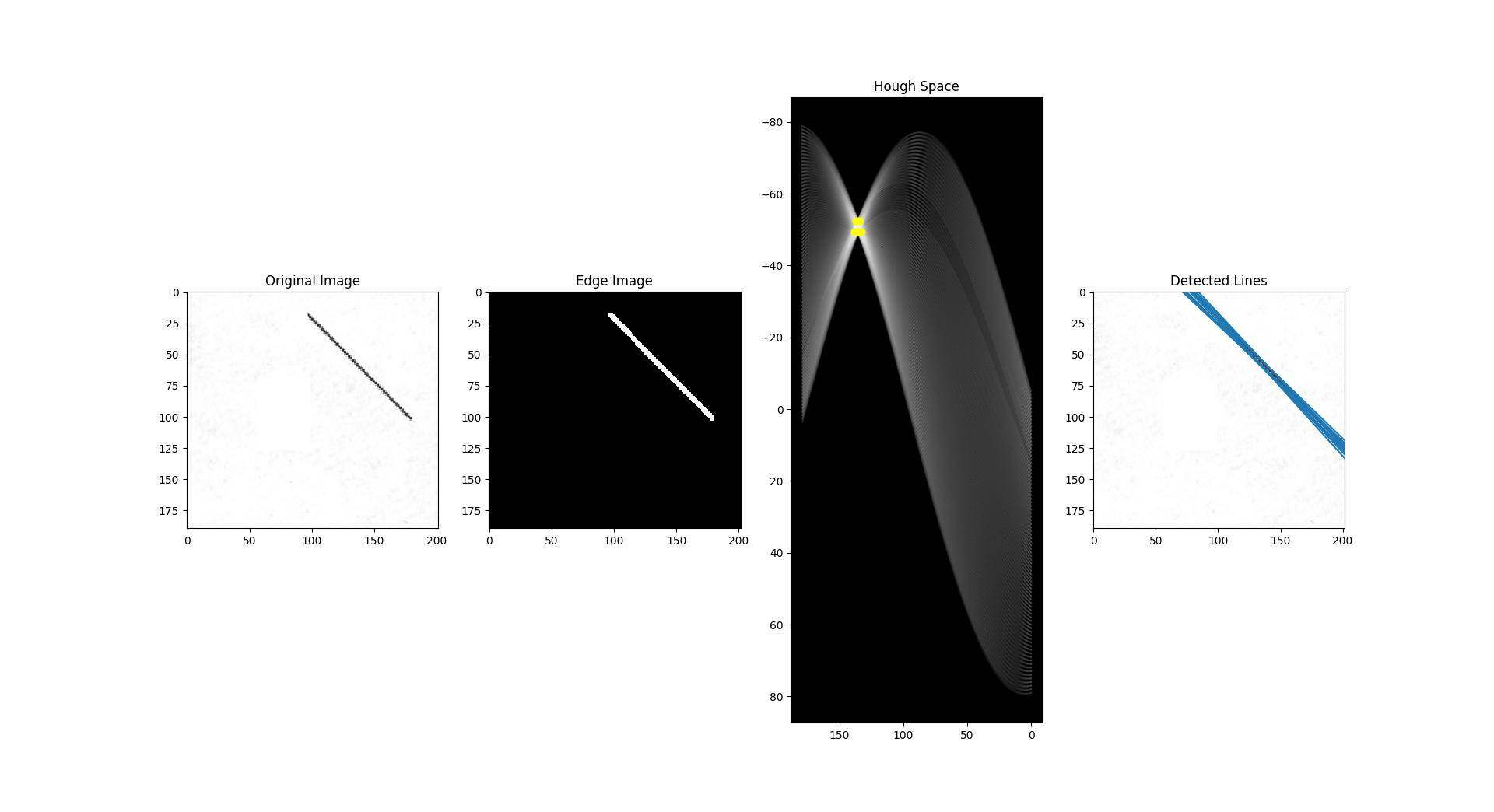## II. 霍夫变换

### 边缘图像Canny 边缘检测算法。来源：https://aishack.in/tutorials/canny-edge-detector/

### 霍夫空间与边缘点到霍夫空间的映射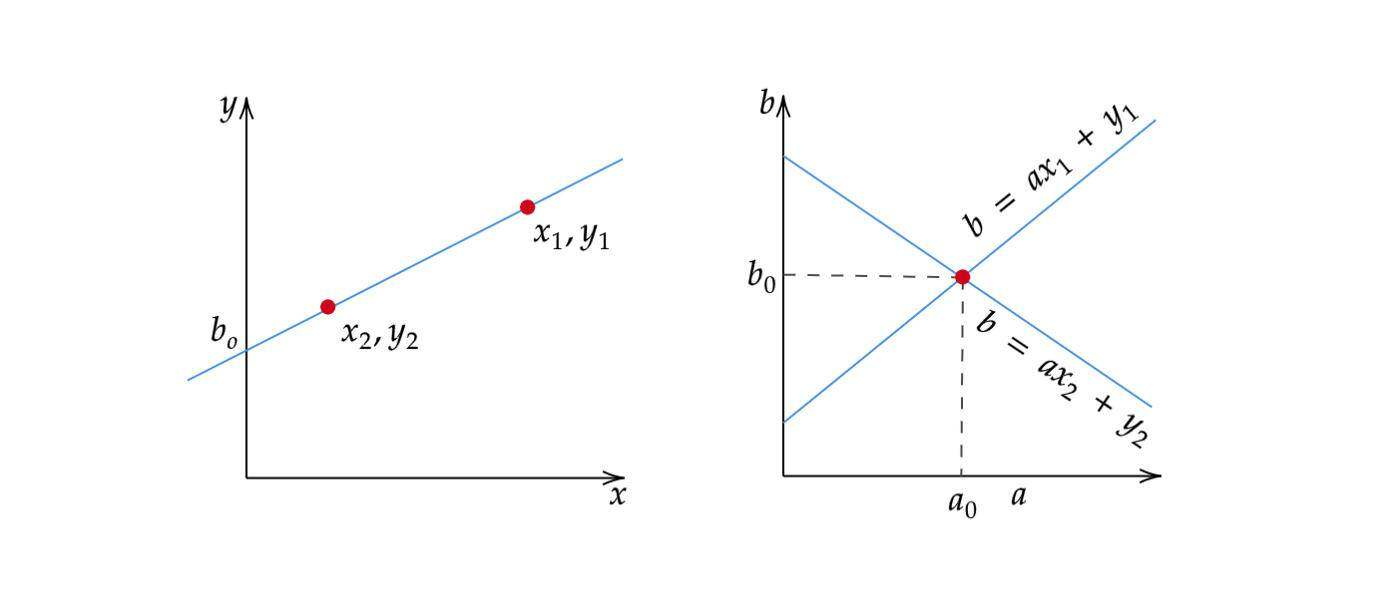### 表示直线的另一种方式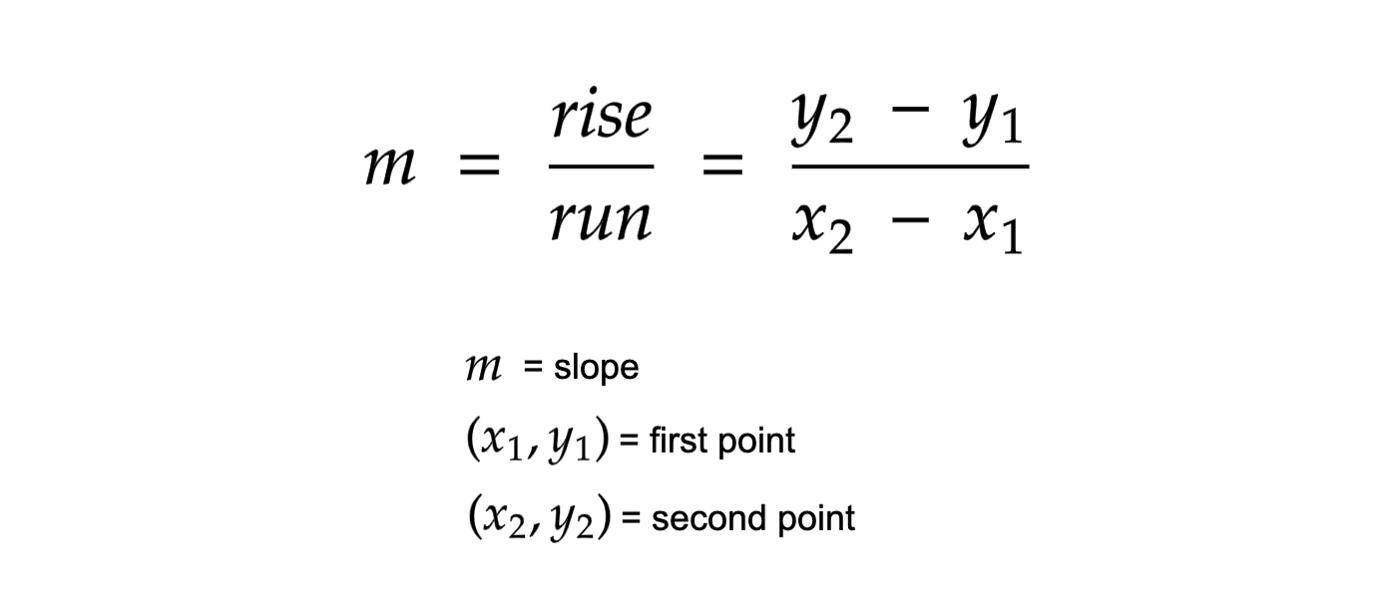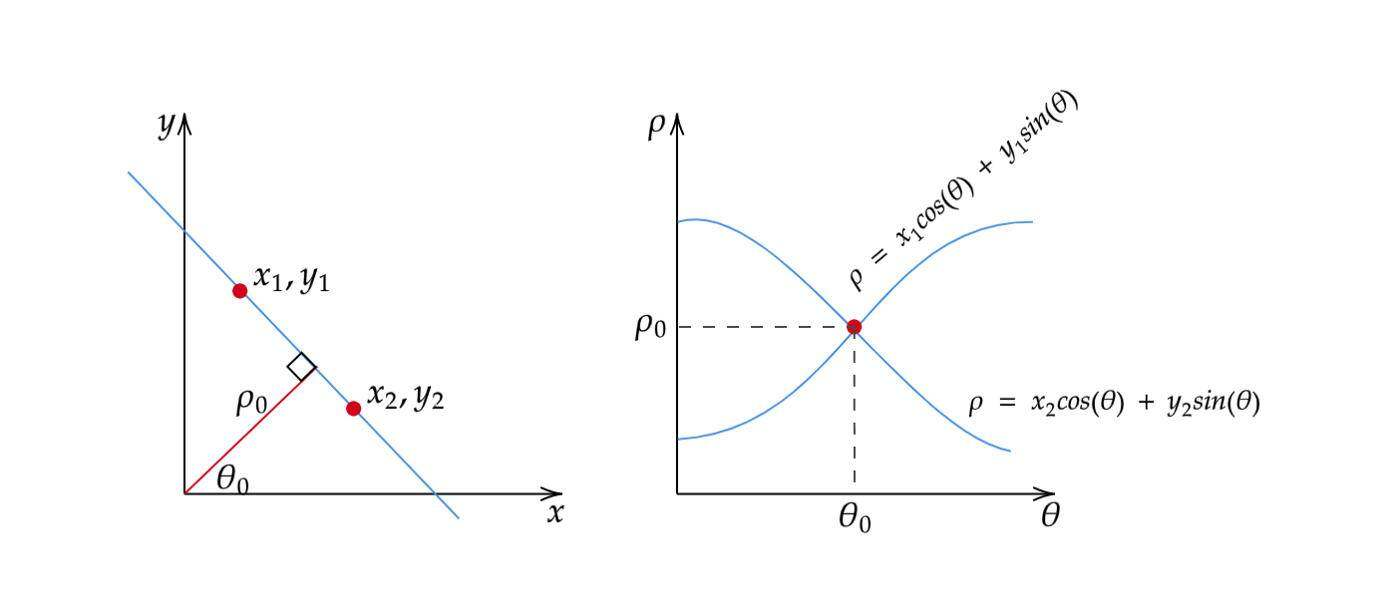### 直线检测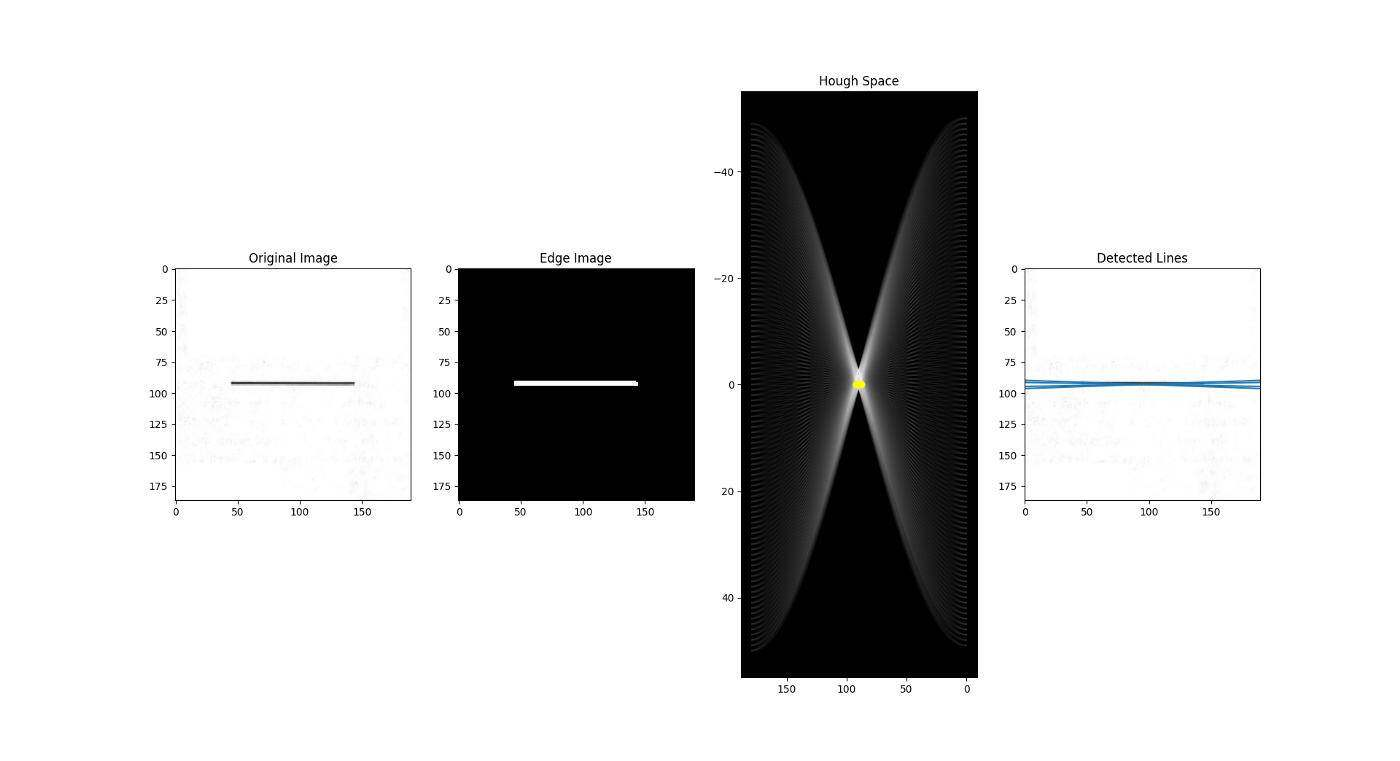## III.算法

1. 确定 的范围。通常， 的范围为 ，其中 是边缘图像的对角线的长度。重要的是要对 的范围进行量化，这意味着这一范围的可能值的数量应该是有限的。

2. 创建一个名为累加器的二维数组，该数组表示具有维数 的霍夫空间，并将其所有制初始化为零。

3. 对原始图像进行边缘检测。这可以用你选择的任何边缘检测算法来完成。

4. 对于边缘图像上的每个像素，检查改像素是否为边缘像素。如果是边缘像素，则循环遍历 的所有可能值，计算相应的 ，在累加器中找到 的索引，并基于这些索引对递增累加器。

5. 循环遍历累加器中的所有制。如果该值大于某一阈值，则获取 的索引，从索引对中获取 的值，然后可以将其转换回 的形式。

## IV. 代码实现

### 非向量化解决方案

import cv2import numpy as npimport matplotlib.pyplot as pltimport matplotlib.lines as mlinesdef line_detection_non_vectorized(image, edge_image, num_rhos=180, num_thetas=180, t_count=220):  edge_height, edge_width = edge_image.shape[:2]  edge_height_half, edge_width_half = edge_height / 2, edge_width / 2  #  d = np.sqrt(np.square(edge_height) + np.square(edge_width))  dtheta = 180 / num_thetas  drho = (2 * d) / num_rhos  #  thetas = np.arange(0, 180, step=dtheta)  rhos = np.arange(-d, d, step=drho)  #  cos_thetas = np.cos(np.deg2rad(thetas))  sin_thetas = np.sin(np.deg2rad(thetas))  #  accumulator = np.zeros((len(rhos), len(rhos)))  #  figure = plt.figure(figsize=(12, 12))  subplot1 = figure.add_subplot(1, 4, 1)  subplot1.imshow(image)  subplot2 = figure.add_subplot(1, 4, 2)  subplot2.imshow(edge_image, cmap="gray")  subplot3 = figure.add_subplot(1, 4, 3)  subplot3.set_facecolor((0, 0, 0))  subplot4 = figure.add_subplot(1, 4, 4)  subplot4.imshow(image)  #  for y in range(edge_height):    for x in range(edge_width):      if edge_image[y][x] != 0:        edge_point = [y - edge_height_half, x - edge_width_half]        ys, xs = [], []        for theta_idx in range(len(thetas)):          rho = (edge_point * cos_thetas[theta_idx]) + (edge_point * sin_thetas[theta_idx])          theta = thetas[theta_idx]          rho_idx = np.argmin(np.abs(rhos - rho))          accumulator[rho_idx][theta_idx] += 1          ys.append(rho)          xs.append(theta)        subplot3.plot(xs, ys, color="white", alpha=0.05)  for y in range(accumulator.shape):    for x in range(accumulator.shape):      if accumulator[y][x] > t_count:        rho = rhos[y]        theta = thetas[x]        a = np.cos(np.deg2rad(theta))        b = np.sin(np.deg2rad(theta))        x0 = (a * rho) + edge_width_half        y0 = (b * rho) + edge_height_half        x1 = int(x0 + 1000 * (-b))        y1 = int(y0 + 1000 * (a))        x2 = int(x0 - 1000 * (-b))        y2 = int(y0 - 1000 * (a))        subplot3.plot([theta], [rho], marker='o', color="yellow")        subplot4.add_line(mlines.Line2D([x1, x2], [y1, y2]))  subplot3.invert_yaxis()  subplot3.invert_xaxis()  subplot1.title.set_text("Original Image")  subplot2.title.set_text("Edge Image")  subplot3.title.set_text("Hough Space")  subplot4.title.set_text("Detected Lines")  plt.show()  return accumulator, rhos, thetasif __name__ == "__main__":  for i in range(3):    image = cv2.imread(f"sample-{i+1}.png")    edge_image = cv2.cvtColor(image, cv2.COLOR_BGR2GRAY)    edge_image = cv2.GaussianBlur(edge_image, (3, 3), 1)    edge_image = cv2.Canny(edge_image, 100, 200)    edge_image = cv2.dilate(        edge_image,        cv2.getStructuringElement(cv2.MORPH_RECT, (5, 5)),        iterations=1    )    edge_image = cv2.erode(        edge_image,        cv2.getStructuringElement(cv2.MORPH_RECT, (5, 5)),        iterations=1    )    line_detection_non_vectorized(image, edge_image)

### 向量化解决方案

import cv2import numpy as npimport matplotlib.pyplot as pltimport matplotlib.lines as mlinesdef line_detection_vectorized(image, edge_image, num_rhos=180, num_thetas=180, t_count=220):  edge_height, edge_width = edge_image.shape[:2]  edge_height_half, edge_width_half = edge_height / 2, edge_width / 2  #  d = np.sqrt(np.square(edge_height) + np.square(edge_width))  dtheta = 180 / num_thetas  drho = (2 * d) / num_rhos  #  thetas = np.arange(0, 180, step=dtheta)  rhos = np.arange(-d, d, step=drho)  #  cos_thetas = np.cos(np.deg2rad(thetas))  sin_thetas = np.sin(np.deg2rad(thetas))  #  accumulator = np.zeros((len(rhos), len(rhos)))  #  figure = plt.figure(figsize=(12, 12))  subplot1 = figure.add_subplot(1, 4, 1)  subplot1.imshow(image)  subplot2 = figure.add_subplot(1, 4, 2)  subplot2.imshow(edge_image, cmap="gray")  subplot3 = figure.add_subplot(1, 4, 3)  subplot3.set_facecolor((0, 0, 0))  subplot4 = figure.add_subplot(1, 4, 4)  subplot4.imshow(image)  #  edge_points = np.argwhere(edge_image != 0)  edge_points = edge_points - np.array([[edge_height_half, edge_width_half]])  #  rho_values = np.matmul(edge_points, np.array([sin_thetas, cos_thetas]))  #  accumulator, theta_vals, rho_vals = np.histogram2d(      np.tile(thetas, rho_values.shape),      rho_values.ravel(),      bins=[thetas, rhos]  )  accumulator = np.transpose(accumulator)  lines = np.argwhere(accumulator > t_count)  rho_idxs, theta_idxs = lines[:, 0], lines[:, 1]  r, t = rhos[rho_idxs], thetas[theta_idxs]  for ys in rho_values:    subplot3.plot(thetas, ys, color="white", alpha=0.05)  subplot3.plot([t], [r], color="yellow", marker='o')  for line in lines:    y, x = line    rho = rhos[y]    theta = thetas[x]    a = np.cos(np.deg2rad(theta))    b = np.sin(np.deg2rad(theta))    x0 = (a * rho) + edge_width_half    y0 = (b * rho) + edge_height_half    x1 = int(x0 + 1000 * (-b))    y1 = int(y0 + 1000 * (a))    x2 = int(x0 - 1000 * (-b))    y2 = int(y0 - 1000 * (a))    subplot3.plot([theta], [rho], marker='o', color="yellow")    subplot4.add_line(mlines.Line2D([x1, x2], [y1, y2]))  subplot3.invert_yaxis()  subplot3.invert_xaxis()  subplot1.title.set_text("Original Image")  subplot2.title.set_text("Edge Image")  subplot3.title.set_text("Hough Space")  subplot4.title.set_text("Detected Lines")  plt.show()  return accumulator, rhos, thetasif __name__ == "__main__":  for i in range(3):    image = cv2.imread(f"sample-{i+1}.png")    edge_image = cv2.cvtColor(image, cv2.COLOR_BGR2GRAY)    edge_image = cv2.GaussianBlur(edge_image, (3, 3), 1)    edge_image = cv2.Canny(edge_image, 100, 200)    edge_image = cv2.dilate(        edge_image,        cv2.getStructuringElement(cv2.MORPH_RECT, (5, 5)),        iterations=1    )    edge_image = cv2.erode(        edge_image,        cv2.getStructuringElement(cv2.MORPH_RECT, (5, 5)),        iterations=1    )    line_detection_vectorized(image, edge_image)

## 参考文献

2020 年 5 月 28 日 16:322857

## 评论

• ###### 模块答疑：软件工程师如何进入人工智能领域？

根据我的经验，我来给你呈现一个软件工程师进入人工智能领域的“学习路线图”，希望可以帮助到想转型进入人工智能领域的同学。

2019 年 2 月 2 日

• ###### 为什么卷积神经网络优于传统机器学习算法?

要真正理解并欣赏深度学习，我们必须知道为什么其他方法失败了，而深度学习却成功了。

• ###### 支持向量机：怎么预测股票市场的涨与跌？

今天，我带你学习支持向量机的原理、应用和优缺点。

2021 年 1 月 13 日

• ###### 虹膜识别技术

本文来自微信京东数科技术说公众号。

• ###### 深度学习人体姿态估计算法综述

人体姿态估计的任务是从包含人体的图片中检测出人体关键点，并恢复人体位姿。随着深度学习的发展，越来越多的深度学习方法被应用到了人体姿态估计任务上来。本文介绍了几个基于深度学习的多人 2D 人体姿态估计算法。

• ###### 只有高中数学基础，要如何掌握数据科学的数学？

原本以为，大学毕业以后就不会再碰数学了。

本文介绍了两种用于目标检测的深度学习库，并对目前主流的目标检测算法进行了分析。

• ###### 加餐 | 前端与图形学

我们打开了一扇新的大门，如果想法再深入一点，我们不需要用图片了，可以直接用代码去渲染了。

2019 年 5 月 2 日

• ###### 如何使用噪声生成复杂的纹理？

你知道怎么在可视化中呈现天上的云朵、水流的波纹以及被侵蚀的土地吗？这些大自然中的图案，其实都能通过随机技巧来实现。

2020 年 7 月 29 日

• ###### 11｜图案生成：如何生成重复图案、分形图案以及随机效果？

你一定见过各种各样的图案，它们有的复杂，有的规律明显，有的看上去比较随机。那你知道这些不同的图案是怎么增强视觉效果的吗？

2020 年 7 月 17 日

• ###### 从感知机到神经网络算法

从机器学习模型角度看，目前最简单的机器学习模型大概就是感知机了，而最火热的机器学习模型则是神经网络。

2019 年 1 月 31 日

6月日更

Linux

rust

#### OpenVINO+微软黑客松比赛项目简介“一键上链”技术大牛教你快速构建链上应用

“一键上链”技术大牛教你快速构建链上应用